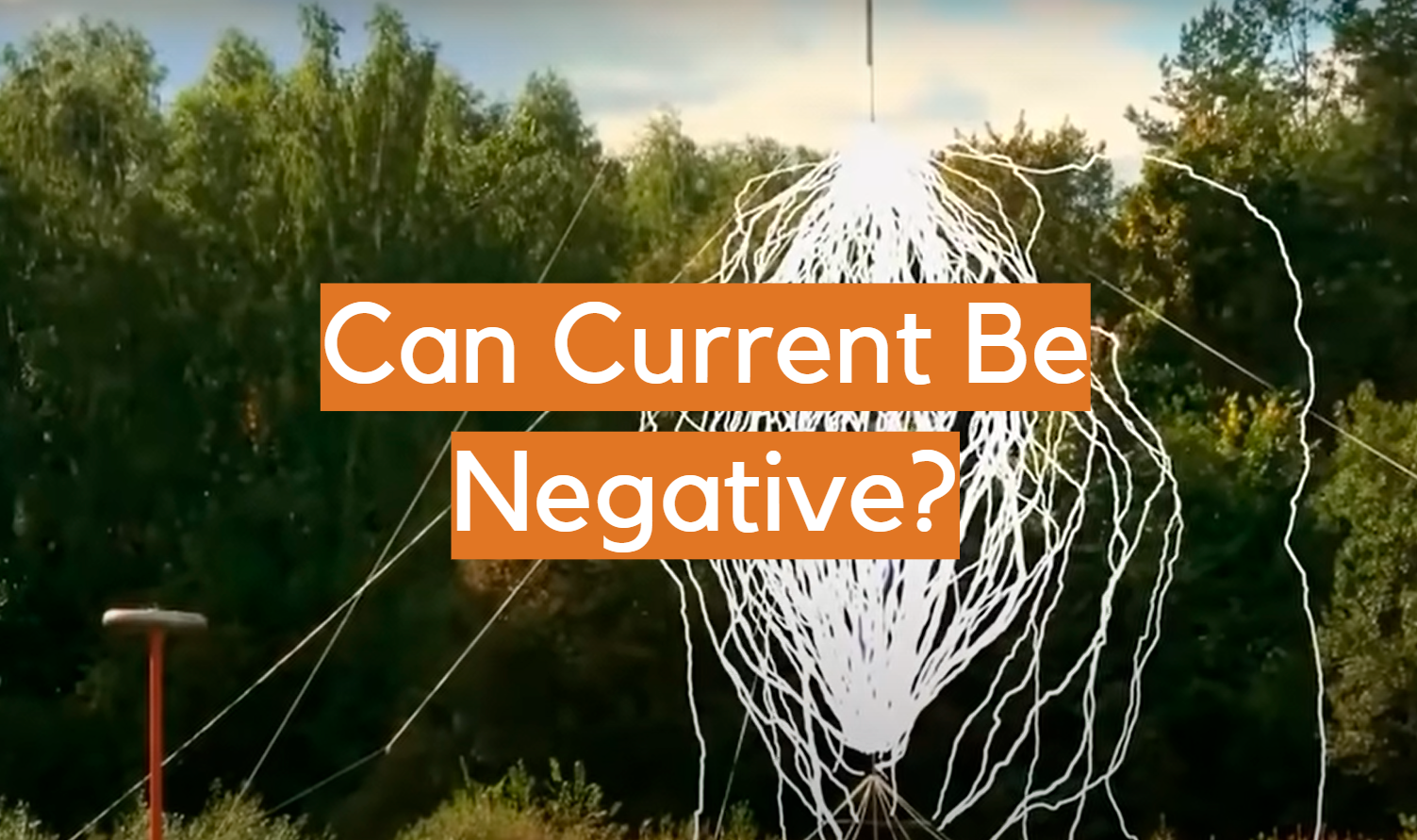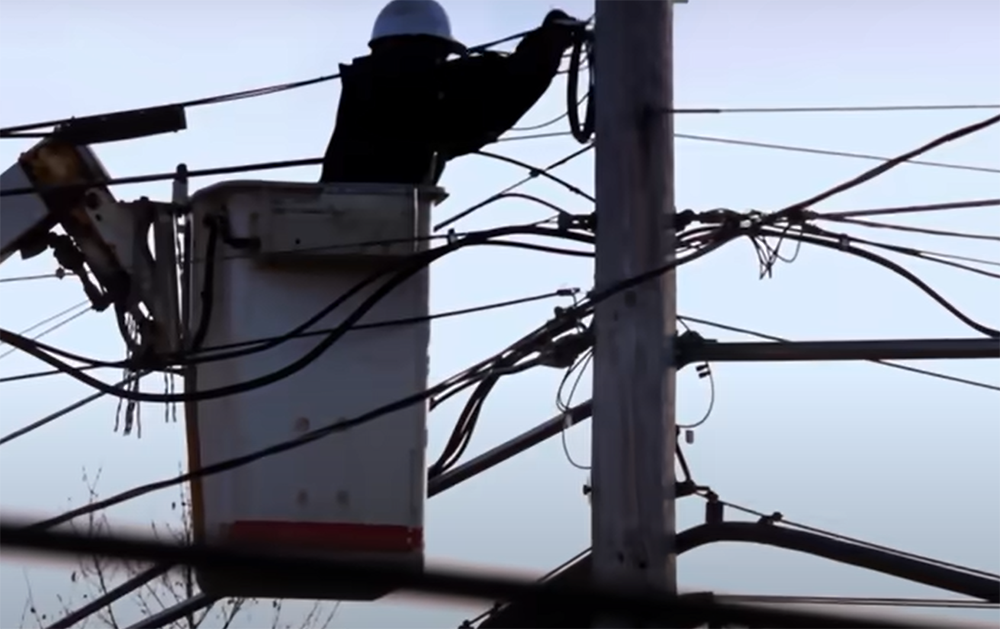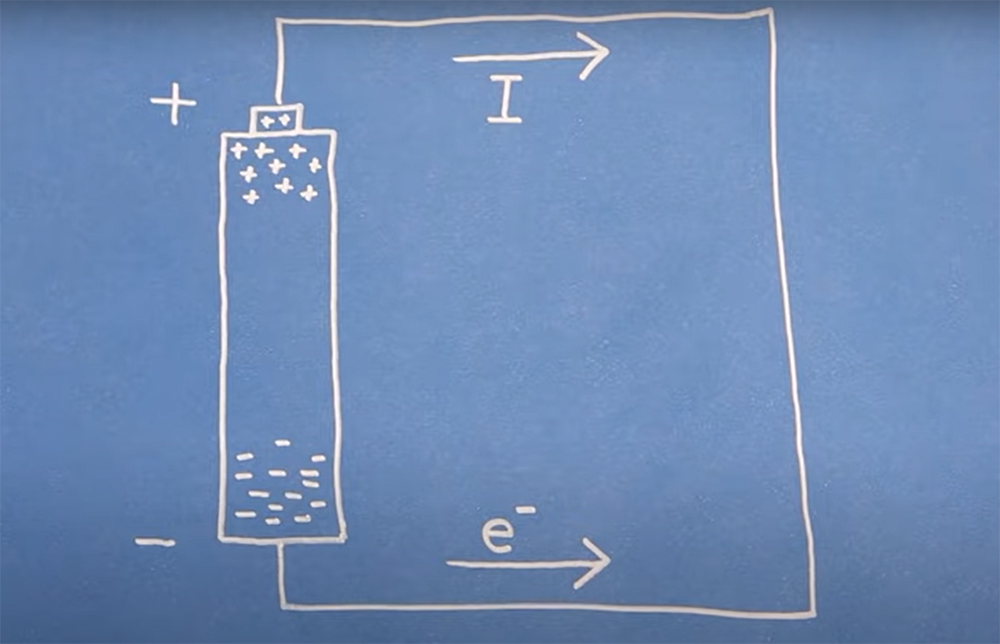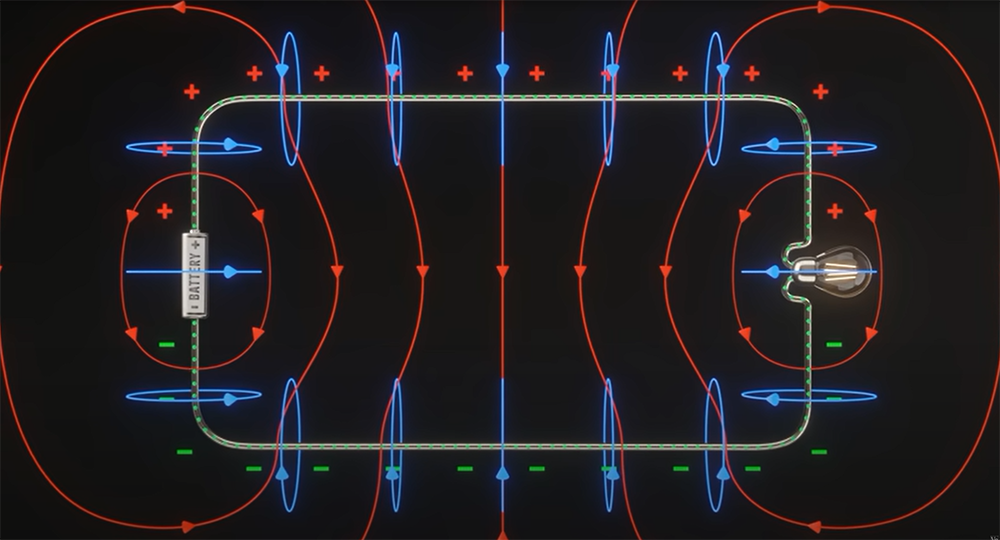# Can Current Be Negative?Electricity is all around us and it’s one of the most important things in our lives. We use it to power our homes, run our appliances, and keep ourselves connected. But what if electricity could have a negative charge? Is that even possible? In this article, we will explore the possibility of negative electric currents and answer some common questions about them. Stay tuned for more information!

## Electric Currents in General

Most people have a basic understanding of electromagnetic current. It is known as the flow of charged particles through a conductor, such as a wire. The particles in question can be electrons, protons, or other charged particles, although not all of them are the same size.The metric unit for electric current is called an ampere. Its short form, “a”, stands for ‘amplitude’ and reflects how much electricity flows through a certain point in one second. 1 ampere = 1 coulomb per second. Coulombs are a unit of measure for electric charge. To explain the connection between two units in more depth, one ampere of electric current means that one coulomb of charge flows through a given point in one second.

Matter is made up of atoms, which are in turn composed entirely from sub-components – proton with a positive charge; electron with a negative charge and their neutral counterparts, neutrons. When these particles flow through conductors then this process creates an electric current! 

## Types of Currents

The two main types of electric current are direct current (DC) and alternating current (AC).

Direct current is a flow of electric charge that travels in one direction only, without alteration. The DC power is mainly used in applications that require low voltage and current, such as charging batteries. In fact, it is present nearly in every household device that uses batteries.

Alternating current is a flow of electric charge that is prone to changing its direction. The most common type of AC is generated by power plants and transmitted through power lines to our homes and businesses.

In general, the term “electric current” describes any flow of electric charge, regardless of its direction. 

Electric currents can be classified further into conventional currents and electron currents. Let’s take a look and discover the main differences between these two terms.

### Conventional electric current

Conventional current is used to describe the direction that positive charges flow. It assumes the current flows from the “+” terminal towards the “-” terminal.

This can be confusing because, as we know, electrons actually flow in the opposite direction (from negative to positive). So what is the basis behind the assumption that the conventional current flows from positive to negative?

The reason has to do with historical convention. When people first started studying electricity, they falsely assumed that electric current was caused by something called “positive charge” flowing through conductors. It wasn’t until later that they realized that it was actually electrons flowing in the opposite direction. However by then, the convention had already been established, and so it has remained to this day.

Even though the conventional current is no longer an accurate description of what’s really happening, it’s still a useful concept because it can be used to predict the behavior of circuits. , , , , , 

### Electron currents

An electron current defines the flow of electrons through a conductor. This is the type of electric current that most people are familiar with nowadays. The electrons flow from the negative terminal of a battery through a wire to the positive terminal.Despite this seemingly up to date definition, in some cases it is still useful to refer to the direction of flow of conventional current. In certain situations, following conventional current is simply more convenient.

It’s important to remember that, despite what you might think first, electric current is not actually a flow of electrons. It’s simply the rate at which charge flows through a conductor. The term “electric current” refers to the movement of any type of charged particles, not just electrons.

The type of electric current that you use is really up to you. If you are just trying to calculate the amount of charge flowing through a conductor, then it doesn’t really matter which one you use as long as consistency is maintained throughout.

However, if you are trying to determine the direction of flow of electric current, then you need to be careful. Remember, the conventional current is the direction of flow of positive charges, while the electron current is the flow of electrons. , , , , , , 

## Which Term is More Used?

The term conventional current was created back when electricity was just discovered, and people didn’t know about the existence of electrons. Benjamin Franklin, who is one of the discoverers of electricity, believed that electric current flowed from positive to negative.

Nowadays, we know that this is not the case – electrons actually flow from negative to positive. However, the term conventional current is still used in some cases. For example, when discussing electrical circuits, it is more convenient to use the direction of conventional current rather than the direction of electron flow.

However, since we now know that it is actually electrons that flow from negative to positive, this term is no longer used much in scientific literature. It is still used in some textbooks, but only for historical reasons and because most equations and even rules are written in terms of conventional current.

The term “electron current” is used more often than “conventional current” in specialized literature. However it is still argued whether or not it’s a preferred term when discussing the direction of flow of electric current in certain circles.

Because of this, it’s important to be familiar with both terms, as you will come across the two of them when reading about electric current. Because of how long the term “conventional current” was used, people are still more familiar with it than they are with “electron current.” , , , , , 

## Does Current Flow Matter?

As we know, current is the flow of electrons around a circuit. But does the direction that these electrons flow actually matter? Well it depends on the device. Based on how devices tolerate and work under different current flow directions, they can be classified as polarized and non-polarized.### Polarized devices

Polarized devices have a positive and negative side, also known as electrical polarity. The current flows in through the positive side and out through the negative. If you try to put the current in through the wrong side on one of these devices, it simply won’t work or will function differently.

### Non-polarized devices

Non-polarized devices don’t have a positive or negative side. The current can flow in either direction and the device will still work in the same way. A good example of this is a resistor. , 

## So Can Electric Current be Negative?

Now that we have a basic understanding of electric current, let’s finally get to the main question and take a look at whether or not they can be negative.

As we know, electric current is the flow of electric charge. And since there are two types of charges – positive and negative – this means that there can be two types of electric current flow: positive and negative.

The direction of the flow depends entirely on…your choice! It’s a convention. You can choose to have the current flow from positive to negative direction, or you can have it flow the opposite way, from negative to positive.

It really doesn’t matter which way you choose, as long as you are consistent with your choice. So if you want to have a negative electric current, go ahead! There is nothing stopping you.

Negative current simply flows in the opposite direction of what you defined as positive, and it will function the same way regardless of definition. 

## FAQ

### Can current flow in both directions at the same time?

The answer to this question is no. Current can only flow in one direction at a time.

This is due to the fact that current is the flow of electrons, and electrons can only flow in one direction. If you were to try and make current flow in both directions at the same time, it would cancel out and there would be no net current flowing.

### What is the difference between conventional current and electron flow?

The terms “conventional current” and “electron flow” are often used interchangeably, but they refer to different things. Conventional current is the direction that positive charges would move if they were free to do so. Electron flow is the actual movement of electrons, which is always in the opposite direction of conventional current.

So why do we use the term “conventional current?” It’s because when Benjamin Franklin first started studying electricity, he assumed that electric currents were carried by positive charges. It wasn’t until later that scientists realized that it was actually electrons flowing in the opposite direction. However, by then the term “conventional current” was already established, and it’s still used today.

### Is current always positive?

The short answer is no, current can be negative. To understand how this is possible, we first need to understand what electric current actually is.

Current is the flow of particles through a conductor. This flow can be either positive or negative. The exact direction though is purely a convention. We always assume that the current is flowing in the direction of the positive charge. But, this is not a rule that is set in stone, and the actual current direction is arbitrary.

If the current is negative, it will flow in the opposite direction to the one you assumed, a very simple definition.

## Conclusion

You may be wondering if electric current can ever be negative. After all, we’ve been talking about positive and negative charges throughout this post and the direction of electron flow is always determined by the polarity of the battery terminals. However, it is important to realize that the direction of a current itself is defined by a convention- regardless of whether or not that corresponds to the actual movement of electrons. So, if the current happens to flow in the opposite direction to what you consider ‘positive’- then yes, you have created a negative current. It’s all just a matter of perspective!